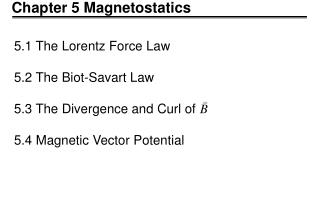Download PresentationChapter 5 Magnetostatics

# Chapter 5 Magnetostatics - PowerPoint PPT PresentationDownload Presentation## Chapter 5 Magnetostatics

- - - - - - - - - - - - - - - - - - - - - - - - - - - E N D - - - - - - - - - - - - - - - - - - - - - - - - - - -
##### Presentation Transcript

1. Chapter 5 Magnetostatics 5.1 The Lorentz Force Law 5.2 The Biot-Savart Law 5.3 The Divergence and Curl of 5.4 Magnetic Vector Potential

2. 5.1.1 Magnetic Fields Source charges Charges induce electric field Test charge

3. 5.1.1

4. EM wave light laser 5.1.2 Magnetic Force Lorentz Force Law Ex.1 Cyclotron motion moment cyclotron frequency relativistic cyclotron frequency microwave relativistic electron cyclotron maser

5. 5.1.2 (2) ~1960 EM : maser [ 1959 J.Schneider ; A.V. Gaponov] ES : space [1958 R.Q. Twiss ] (1976) K.R. Chu & J.L. Hirshfield : physics in gyrotron/plasma 1978 C.S. Wu & L.C. Lee : EM in space ( ) 1986 K.R. Chen & K.R. Chu : ES in gyrotron relativistic ion cyclotron instability 1993 K.R. Chen ES in Lab. plasma [fusion ( EM ? Lab. & space plasmas ? )]

6. 5.1.2 (3) Ex.2 Cycloid Motion assume

7. 5.1.2 (4)

8. 5.1.2 (5)

9. 5.1.2 (6) Magnetic forces do not work

10. 5.1.3 Currents The current in a wire is the charge per unit time passing a given point. Amperes 1A = 1 C/S The magnetic force on a segment of current-carrying wire

11. 5.1.3 (2) surface current density the current per unit length-perpendicular-to-flow (mobile) The magnetic force on a surface current is

12. 5.1.3 (3) volume current density The current per unit area-perpendicular-to-flow The magnetic force on a volume current is

13. 5.1.3 (4) Ex. 3 Sol. Ex. 4 (a) what is J ? (uniform I) Sol.

14. 5.1.3 (5) (b) For J = kr, find the total current in the wire. Sol.

15. 5.1.3 (6) relation? Continuity equation (charge conservation)

16. 5.2.1 Steady Currents Stationary charges constant electric field: electrostatics Steady currents constant magnetic field: magnetostatics No time dependence

17. 5.2.2 The Magnetic Field of a Steady Current Biot-Savart Law: for a steady line current Permeability of free space Biot-Savart Law for surface currents Biot-Savart Law for volume currents for a moving point charge

18. 5.2.2 (2) Solution: In the case of an infinite wire,

19. 5.2.2 (3) The field at (2) due to is The force at (2) due to is Force? The force per unit length is

20. 5.3.2 The Divergence and Curl of Biot-savart law

21. 5.3.2 for steady current To where Ampere’s law in differential form

22. 5.3.3 Applications of Ampere’s Law Ampere’s Law in differential form Ampere’s Law in integral form Electrostatics: Coulomb Gauss Magnetostatics: Bio-Savart Ampere The standard current configurations which can be handled by Ampere's law: • Infinite straight lines • Infinite planes • Infinite solenoids • Toroid

23. 5.3.3 (2) ? Ex.7 symmetry Ex.8

24. 5.3.3 (3) Ex.9 loop 1. loop 2.

25. 5.3.3 (4) Ex.10 Solution:

26. 5.3.3 (5)

27. M.S. : a constant-like vector function 5.4.1 The Vector Potential E.S. : Gauge transformation is a vector potential in magnetostatics If there is that , can we find a function to obtain with

28. 5.4.1 (2) Ampere’s Law if

29. 5.4.1 (3) Example 11 A spiring sphere Solution: For surface integration over easier

30. 5.4.1 (4)

31. 5.4.1 (5)

32. 5.4.1 (6) if R > S if R < S

33. 5.4.1 (7)

34. 5.4.1 (8) Note: is uniform inside the spherical shell

35. 5.4.2 Summary and Magnetostaic Boundary Conditions

36. 5.4.2 (2)

37. 5.4.2 (3)

38. 5.4.3 Multipole Expansion of the Vector Potential line current =0 monopole dipole

39. 5.4.3(2)

40. 5.4.3(3) Ex. 13

41. 5.4.3(4) Field of a “pure” magnetic dipole Field of a “physical” magnetic dipole